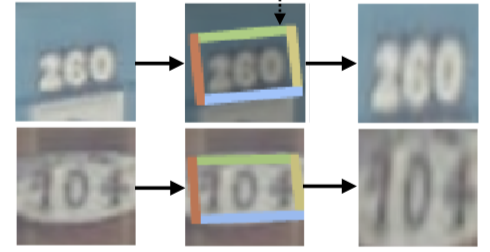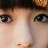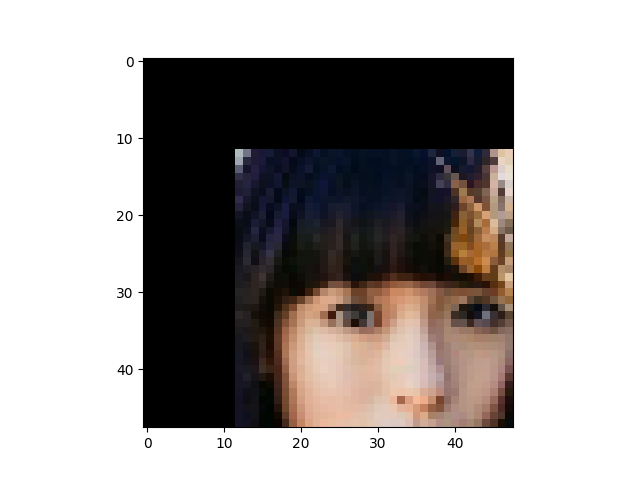# How can I use stn as crop and resize?

I want to use stn to crop a patch and resize to the specific size.This is STN, we can draw and visualize the cropped bounding box.

given the transformation matrix, similar effect can be done in opencv

original image:``````src_rgb = cv2.imread('./0001_01.jpg')
Affine_Mat_w = [1., 0, -32]
Affine_Mat_h = [0, 1., -32]
M = np.c_[ Affine_Mat_w, Affine_Mat_h].T
res = cv2.warpAffine(src_rgb, M, (48, 48))
``````

crop and resizeAlthough pytorch provide grid_sample and affine_grid, but it does not act like opencv

``````src_rgb = Image.open('./0001_01.jpg')
trans = transforms.ToTensor()
trans1 = transforms.ToPILImage()
grid = torch.nn.functional.affine_grid(M, (1, 3, 48, 48))
y = torch.nn.functional.grid_sample(trans(src_rgb).unsqueeze(dim=0).float(), grid.float())
plt.imshow(convert_image_np(y))
plt.show()
``````

it’s blur and leave many black area.Any idea?

Have you figure out how to crop the image with `F.affine_grid` ?

you can use crop_and_resize from kornia